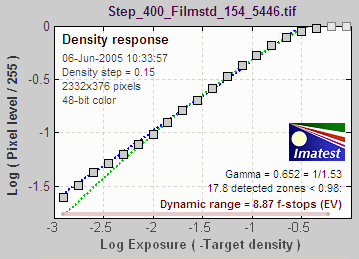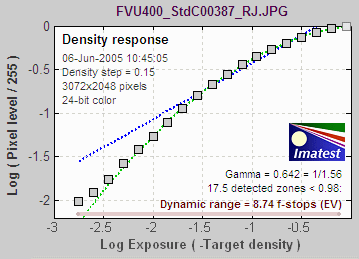# Gamma, Chart Contrast and MTF Calculations

Gamma (the average slope of log pixel levels as a function of log exposure for light through dark gray tones) is used, per the ISO 12233 standard, to linearize the input data, i.e., to remove the gamma encoding applied by the camera or RAW converter. Gamma defaults to 0.5 = 1/2, which is typical of digital cameras, but may be affected by camera or RAW converter settings.

#### Imatest Settings

For accurate calculation of edge-SFR from using Imatest’s SFRplus, eSFR ISO, SFRreg, Checkerboard, or SFR modules, it’s important to use a proper gamma for sharpness calculations.

If the chart contrast is known and is ≤10:1 (medium or low contrast), you can enter the contrast in the Chart contrast (for gamma calc.) box, then check the Use for MTF checkbox. Gamma will be then be calculated from the chart and displayed in the Edge/MTF plot.

#### Measuring Gamma

If chart contrast is not known you should measure gamma by obtaining the OECF (Opto-Electric Conversion Function) per the ISO 14524 standard.  This can be obtained using an image of a grayscale stepchart and running Colorcheck, Stepchart , Multicharts (interactive), or Multitest. A nominal value of gamma should be entered, even if the value of gamma derived from the chart (described above) is used to calculate MTF.

#### Errors in Gamma

Small errors in gamma have a minor effect on MTF measurements (a 10% error in gamma results in a 2.5% error in MTF50 for a normal contrast target). Gamma should be set to 0.45 when dcraw is used to convert RAW images into sRGB or a gamma=2.2 (Adobe RGB) color space. If gamma is set to less than 0.3 or greater than 0.8, the background will be changed to pink to indicate an unusual (possibly erroneous) selection.

### Technical Details1. Capture One LE set to Film standard
(the default). Gamma = 0.679.2. Capture One LE, Linear response. Gamma =
0.508. Recommended for SFR runs.3. Canon FVU set to Standard contrast.
Gamma = 0.642.

Gamma is the exponent of the equation that relates image pixel level to luminance. For a monitor or print,

Output luminance = (pixel level)gamma_display

When the raw output of the image sensor, which is linear, is converted to image file pixels for a standard color space, the approximate inverse of the above operation is applied.

pixel level = (RAW pixel level)gamma_camera ~= exposuregamma_camera

The total system gamma is gamma_display * gamma_camera. Standard values of display gamma are 1.8 for older color spaces used in the Macintosh and 2.2 for color spaces used in Windows, such as sRGB (the default) and Adobe RGB (1998).

The three curves on the right, produced by Stepchart for the Canon EOS-10D, show how Gamma varies with RAW converter settings.In characteristic curves for film and paper, which use logarithmic scales (e.g., density (–log10(absorbed light) vs. log10(exposure)), gamma is the average slope of the transfer curve (excluding the “toe” and “shoulder” regions near the ends of the curve), i.e.,

Gamma is contrast.

To obtain the correct MTF, Imatest must linearize the pixel levels— the camera’s gamma encoding must be removed. That is the purpose of Gamma in the SFR input dialog box, which defaults to 0.5, typical for digital cameras. It can, however, vary considerably with camera and RAW converter settings, most notably contrast.

Characteristic curves for the Canon EOS-10D with three RAW converter settings are shown on the right. Gamma deviates considerably from 0.5. Gamma = 0.679 could result in a 9% MTF50 error. For best accuracy we recommend measuring gamma using Colorcheck or Stepchart, which provides slightly more detailed results.

Confusion factor: Digital cameras rarely apply an exact gamma curve: A “tone reproduction curve” (an “S” curve) is often superposed on the gamma curve to extend dynamic range while maintaining visual contrast. This reduces contrast in highlights and (sometimes) deep shadows while maintaining or boosting it in middle tones. You can see it in curves 1 and 3, on the right. For this reason, “Linear response” (where no S-curves is applied on top of the gamma curve) is recommended for SFR measurements.

The transfer function may also be adaptive: camera gamma may be higher for low contrast scenes than for contrasty scenes. This can cause headaches with SFR measurements. But it’s not a bad idea generally; it’s quite similar to the development adjustments (N-1, N, N+1, etc.) in Ansel Adams’ zone system. For this reason it’s not a bad idea to place a Q-13 or Q-14 chart near the slanted edges.